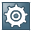Show TOC

###Key Figures in Product Cost by PeriodThe following cost objects are used in Product Cost by Period:

You can display the key figures:

Which key figures can be analyzed depends on the object. For general information on the key figures in Cost Object Controlling, see the following:

Key Figures in Cost Object Controlling

##### Functions of Cost Analysis

In cost analysis, you determine which key figures you analyze in Product Cost by Period. Cost analysis reports use the functionality of the ABAP List Viewer while offering additional functions in cost analysis. You can define layouts in cost analysis containing the key figures that you want to analyze. The column selection for the layouts contains different field groups (column lists) that separate the key figures into logical groups.

##### You can analyze the following for product cost collectors:
• Key figures that result from actual postings (such as total actual costs, actual fixed costs, actual variable costs, and the yield)

• Work in process at target cost (see also:Work in Process in Product Cost by Period)

• Data generated by variance calculation. Examples:

• Target costs

• Control costs

• Individual variance categories

• Absolute and percentage variances

You cannot analyze planned costs for product cost collectors because no planned costs are updated for product cost collectors in preliminary costing. You can analyze the results of preliminary cost estimates for product cost collectors in the information system of Product Cost Planning in the reports for material cost estimates with a quantity structure.

##### You can analyze the following for cost object hierarchies:
• Key figures that result from actual postings (such as total actual costs, actual fixed costs, actual variable costs, and the yield)

• Planned costs, provided that the planned costs were updated on the manufacturing orders assigned to a cost object hierarchy (such as summarization of the planned costs that were calculated in preliminary costing for the manufacturing orders assigned to a cost object hierarchy). Planned costs cannot be displayed for product cost collectors that are assigned to a cost object hierarchy.

• Plan/actual comparisons

• Work in process at target costs, provided that work in process was calculated for the orders assigned to the cost object hierarchy No work in process can be determined for cost object hierarchies.

• Data generated by variance calculation Examples:

• Target costs

• Control costs

• Individual variance categories

• Absolute and percentage variances

Key Figures in Cost Object Controlling

Actual Cost Distribution in Cost Object Hierarchies

Work in Process in Product Cost by Period

Types of Variance Calculation

Example: Value Flow with Work in Process at Target Costs

Scrap Variance

Target Cost Versions in Variance Calculation

Calculation of Target Costs

Calculation of Control Costs

Variances with a Valuated Sales Order Stock

Periodic Variance Calculation: Value FlowNote

Summarized Analysis with Summarization Hierarchy

If variances are calculated for an order (product cost collector or manufacturing order), the system sets the status VCAL (variances calculated) on the order. To assure comparability, you should only summarize objects for which variances have been calculated. Use the status selection profile in the required data collection. Select the orders for which the status VCAL is active.

If objects with the settlement type periodic or full are summarized together and analyzed by period, this should be taken into account when the report is interpreted.

Differences in the Summarization Methods

End of the note.

##### Product Drilldown Reports

See the following sections for information on product drilldown reports:

##### Object Lists

See the following sections for information on object lists:

##### Object Comparisons

See the following sections for information on object comparisons:

You can display summarized data for cost object hierarchies in the report categories Object Lists and Detailed Reports.